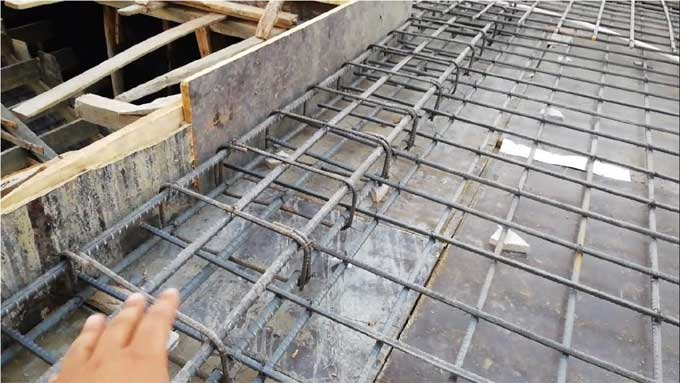# A Guide of RCC Roof Slab Construction CostReinforced cement concrete, or RCC, is commonly referred to as concrete in the construction industry. The concrete reinforced with steel bars and cement provided a high level of strength is one of the primary elements of modern structural design.

Building materials such as RCC is widely used in the construction industry. You will calculate the construction costs when you are using structural elements in your home or office.

### Key Details of RCC Roof Slab Reinforcement

According to built-in structural design standards for modern buildings, a flat slab is created instead of a beam in the RCC. Including the beams would effectively transfer a load from the slab to the columns, thereby making the structure more durable.

Depending on the type of column, the cross-section dimension can be 9" X 9" or 9" X 12". The minimum steel bars for the column must be 12 MM in diameter and the stirrups must be 8 MM in diameter. It is recommended that the beam size not be less than 9" X 9" with a minimum of 4 steel bars, to include 2 12MM thick bars on the bottom and 2 10MM thick bars on top.

The slab thickness should not be less than 5 inches, since electrical pipes and internal wiring can make the slab depth less than 5 inches at some places. For an RCC roof, reinforcement details fall into two categories:

Main Steel Bars

Main steel bars are 8 millimeters and distribution steel bars are 6 millimeters in one way slabs.

Double-way Slab Bars

Double-way slab 8MM steel bars on the main part and 8MM steel bars on the distribution part. In addition to preventing cheating, knowing the above standards will help you calculate the construction costs ahead of time for RCC.

### RCC Roof Slab Construction Cost Calculation

The concrete must first shape to make concrete reinforced with reinforcing bars, followed by a cage of reinforcement, and finally filled with the right amount of concrete mixture and allowed to cure for one month.

If you use a small contractor, you can complete the entire process. But you have to estimate the construction costs to determine whether the quotation offered by the contractor is acceptable. It depends on a lot of factors, such as:

A. A slab's thickness.
B. Slabs are two-way or one-way.
C. Methods and techniques of construction.
D. Columns and beams must be thick enough.

Calculating steel roof slabs using RCC can provide a rough estimate. To figure out the exact amount the construction of a slab of RCC roof will cost, let's look at an example.

A 25X20-square RCC roof slab would be appropriate. A construction project with a size of 1,280 sq. ft. and a concrete mix ratio of 1:2:4 will require calculations of:

A. Area covered by concrete slabs
B. Calculation of the concrete cost
C. Worker's compensation
D. Cost of shuttering
E. Cost of steel

The slab will contain approximately 60 to 65 kg of steel per cubic meter.

? Assuming an area of 25' x 20' for the concrete slab
? It should be converted into cubic meters
? The following equation equals (25x.3048) x (20x.3048)
? This is 4.6451 square meters, so 7.62 x 6.096 equals 46.451 square meters
? Roof slabs must be 125mm thick at a minimum.
? Therefore, 46.451 times .125 = 5.706 Cumulative User Minutes
? Premix concrete costs approximately 3000 per cubic meter;
? Furthermore, Mason - 3 no, MC - 4 no labor charges
? The product of (3x500)+(4x350) Is equal to 2900

? The cost of shuttering is 500
? The concrete cost is therefore (5.806?3000) + 2900+ 500. The amount is Rs 20819.